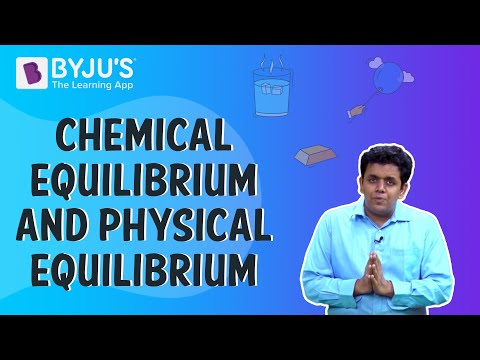# Equilibrium Class 11 Notes - Chapter 7

Equilibrium is attained when molecules leaving the liquid to vapour equals the molecules returning to the liquid from vapour. Kc is the equilibrium constant. It is defined as the concentration of products divided by the concentration of reactants where each term is raised to the stoichiometric coefficient.
Kc = [C]c[D]d/[A]a[B]b## Le Chatelier’s Principle

It states that change in any factor such as concentration, pressure, temperature, etc. causes the equilibrium to shift in such a direction to counteract or reduce the effect of a change.

## Electrolytes

Electrolytes are substances which conduct electricity in aqueous solution. Some examples of electrolytes are bases, salts, and acids. The electricity conducted in aqueous solution is due to cations and anions produced by the ionization or dissociation of electrolytes in the solution.

## Lewis Acid and Base

A Lewis acid is a species containing an empty orbital which can accept an electron pair (an electrophile) from a Lewis base and forms a Lewis adduct.

A Lewis base is a species having a filled orbital which contains an electron pair that will not be involved in bonding but might form a dative bond with Lewis acid.

Also Read: Lewis Acids and Bases

### Important Questions

1. While writing the equilibrium constant expression, you can ignore pure liquids and solids. Why? Explain.

2. Tick mark Lewis acids from the following

H2O, H+, NH+4 and BF3

3. Below are Brönsted bases. Write the conjugate acids for the same.

NH2, HCOO, NH3. Cupric chlorate and sodium iodate having an equal volume of 0.002M. Will the precipitation of copper iodate will occur or not?

4. Cupric chlorate and sodium iodate having an equal volume of 0.002M. Will the precipitation of copper iodate will occur or not?

5. Calculate the solubilities of

a) barium chromate

b) ferric hydroxide

d) mercurous iodide

e) silver chromate

At 300K from their solubility product constant. Also, calculate the molarities of the individual ions.

#### For More Questions On Equilibrium, Watch The Below Video: